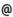## "Babes-Bolyai" University of Cluj-Napoca Faculty of Mathematics and Computer Science

 Applied functional analysis
 Code Semes-ter Hours: C+S+L Credits Type Section MO261 1 2+1+1 8 compulsory Analiză Reală şi Complexă - în limba engleză
 Teaching Staff in Charge
 Prof. COBZAS Stefan, Ph.D., scobzasmath.ubbcluj.ro
 Aims To present some results from the geometric banach space theory with applications to the differentiability of convex functions and to holomorphic vector functions.
 Content 1. Complements of Banach space theory Support functionals - applications to best approximation, Bishop-Phelps subreflexivity theorem and James reflexivity theorem. Weakly compact sets - Eberlein-Shmulian and James-Price theorems. Goldstein theorem on the weak star density of a Banach space in its bidual. Weakly compact operators and Gantmacher theorem. 2. Geometric theory of normed spaces Strictly convex, locally uniformly convex and uniformly convex normed spaces. The reflexivity of uniformly convex Banach spaces. The uniform convexity of the spaces Lp and their moduli of uniform convexity. Characterizations of extreme and smooth points of the unit balls in concrete Banach spaces. Banach spaces with normal structure and fixed point theorems for nonexpansive mappings. 3. Differential calculus in normed spaces Gateaux and Frechet differentials and relations between them. Calculus rules in normed spaces. The differential of the inverse mapping, the theorem of local inversion and the implicit function thoerem. Bilinear mappings anf second oreder differentials. Multilinear mappings and higher order differentials. Taylor formula. Conditioned extrema and Liusternik theorem. Convex functions - continuity properties, Gateaux differentiability properties (Mazur theorem), Frechet differentiability properties. Smoothness properties of normed spaces- Gateaux and Frechet differentiabilty of the norm, smooth spaces, locally uniformly smooth and uniformly smooth spaces, Asplund spaces, examples. Banach-valued functions with bounded variation and Riemann-Stieltjes integral. Holomorphic and analytic Banach-valued functions. The equivalence of weak and strong holomorphy. Applications to the spectral calculus of linear operators. 4. Hahn-Banach type theorem for linear operators Finite intersection property and Nachbin theorem on the norm-preserving extension of continuous linear operators. The characterization of Banach spaces having the finite intersection property. Kantorovich and Krein theorems on the extension of linear operators on ordered spaces. Equivalence of Hahn-Banach theorem and the existence of supremum in ordered vector spaces.
 References 1. DEVILLE R., GODEFROY G., ZIZLER V.: Smoothness and Renormings in Banach Spaces.New York: John Wiley, 1993. 2. FABIAN M. et al.: Functional Analysis and Infinite-Dimensional Geometry. Berlin - New York: Springer Verlag, 2001. 3. GILES J. R.: Convex Analysis with Applications in the Differentiation of Convex Functions. London: Pitman, 1982. 5. HOLMES R. B.: Geometric Functional Analysis. Berlin: Springer-Verlag, 1975. 6. KANTOROVICI L. V., AKILOV G. P.: Analiza functionala. Bucuresti: Editura Stiintifica si Enciclopedica, 1986. 7. KUTATELADZE S. S.: Fundamentals of Functional Analysis. Dordrecht: Kluwer Academic Publishers, 1995. 8. MUNTEAN I.: Analiza functionala. Capitole speciale. Cluj-Napoca: Universitatea Babes-Bolyai, 1990. 9. RUDIN W.: Functional Analysis. New York: McGraw Hill, 1973. 10. YOSIDA K.: Functional Analysis. Berlin: Springer-Verlag, 1995.
 Assessment Exam.